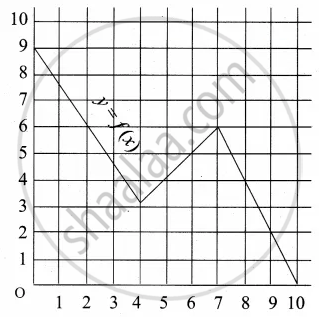Tamil Nadu Board of Secondary EducationSSLC (English Medium) Class 10th

# A graph representing the function f(x) is given in it is clear that f(9) = 2 For what value of x is f(x) = 1? - Mathematics

Graph
Sum

A graph representing the function f(x) is given in it is clear that f(9) = 2For what value of x is f(x) = 1?

#### Solution

When f(x) = 1 the value of x is 9.5

Concept: Functions
Is there an error in this question or solution?